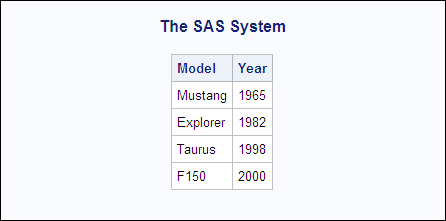# Determining the Observation Boundary to Avoid Concatenated Data

This example imports an XML document that illustrates how to determine the observation boundary so that the result is separate observations and not concatenated data.
The observation boundary translates into a collection of rows with a constant set of columns. Using an XMLMap, you determine the observation boundary with the TABLE-PATH element by specifying a location path. The end tag for the location path determines when data is written to the SAS data set as an observation.
Identifying the observation boundary can be tricky due to sequences of start-tag and end-tag pairing. If you do not identify the appropriate observation boundary, the result could be a concatenated data string instead of separate observations. This example illustrates pairing situations that can cause unwanted results.
For the following XML document, an XMLMap is necessary to import the file successfully. Without an XMLMap, the XML engine would import a data set named FORD with columns ROW0, MODEL0, YEAR0, ROW1, MODEL1, YEAR1, and so on.
```<?xml version="1.0" ?>
<VEHICLES>
<FORD>
<ROW>
<Model>Mustang</Model>
<Year>1965</Year>
</ROW>
<ROW>
<Model>Explorer</Model>
<Year>1982</Year>
</ROW>
<ROW>
<Model>Taurus</Model>
<Year>1998</Year>
</ROW>
<ROW>
<Model>F150</Model>
<Year>2000</Year>
</ROW>
</FORD>
</VEHICLES>```
Looking at the above XML document, there are three sequences of element start tags and end tags: VEHICLES, FORD, and ROW. If you specify the following table location path and column locations paths, the XML engine processes the XML document as follows:
```<TABLE-PATH syntax="XPath"> /VEHICLES/FORD </TABLE-PATH>
<PATH syntax="XPath"> /VEHICLES/FORD/ROW/Model </PATH>
<PATH syntax="XPath"> /VEHICLES/FORD/ROW/Year </PATH>```
1. The XML engine reads the XML markup until it encounters the <FORD> start tag, because FORD is the last element specified in the table location path.
2. The XML engine clears the input buffer and scans subsequent elements for variables based on the column location paths. As a value for each variable is encountered, it is read into the input buffer. For example, after reading the first ROW element, the input buffer contains the values `Mustang` and `1965`.
3. The XML engine continues reading values into the input buffer until it encounters the </FORD> end tag, at which time it writes the completed input buffer to the SAS data set as an observation.
4. The end result is one observation, which is not what you want.
Here is the PRINT procedure listing output showing the concatenated observation. (The data in the observation is truncated due to the LENGTH element.)
PRINT Procedure Output Showing Unacceptable FORD Data Set
```                                 The SAS System                                1

Model                   Year

Mustang Explorer Tau    1965```
To get separate observations, you must change the table location path so that the XML engine writes separate observations to the SAS data set. Here are the correct location paths and the process that the engine would follow:
```<TABLE-PATH syntax="XPath"> /VEHICLES/FORD/ROW </TABLE-PATH>
<PATH syntax="XPath"> /VEHICLES/FORD/ROW/Model </PATH>
<PATH syntax="XPath"> /VEHICLES/FORD/ROW/Year </PATH>```
1. The XML engine reads the XML markup until it encounters the <ROW> start tag, because ROW is the last element specified in the table location path.
2. The XML engine clears the input buffer and scans subsequent elements for variables based on the column location paths. As a value for each variable is encountered, it is read into the input buffer.
3. The XML engine continues reading values into the input buffer until it encounters the </ROW> end tag, at which time it writes the completed input buffer to the SAS data set as an observation. That is, one observation is written to the SAS data set that contains the values `Mustang` and `1965`.
4. The process is repeated for each <ROW> start-tag and </ROW> end-tag sequence.
5. The result is four observations.
Here is the complete XMLMap syntax:
```<?xml version="1.0" ?>
<SXLEMAP version="2.1" name="path" description="XMLMap for path">
<TABLE name="FORD">
<TABLE-PATH syntax="XPath"> /VEHICLES/FORD/ROW </TABLE-PATH>
<COLUMN name="Model">
<DATATYPE> string </DATATYPE>
<LENGTH> 20 </LENGTH>
<TYPE> character </TYPE>
<PATH syntax="XPath"> /VEHICLES/FORD/ROW/Model </PATH>
</COLUMN>
<COLUMN name="Year">
<DATATYPE> string </DATATYPE>
<LENGTH> 4 </LENGTH>
<TYPE> character </TYPE>
<PATH syntax="XPath"> /VEHICLES/FORD/ROW/Year </PATH>
</COLUMN>
</TABLE>
</SXLEMAP>
```
The following SAS statements import the XML document and specify the XMLMap. The PRINT procedure verifies the results.
```filename PATH 'c:\My Documents\XML\path.xml';
filename MAP 'c:\My Documents\XML\path.map';

libname PATH xmlv2 xmlmap=MAP;

proc print data=PATH.FORD noobs;
run;```
PRINT Procedure Output Showing FORD Data Set